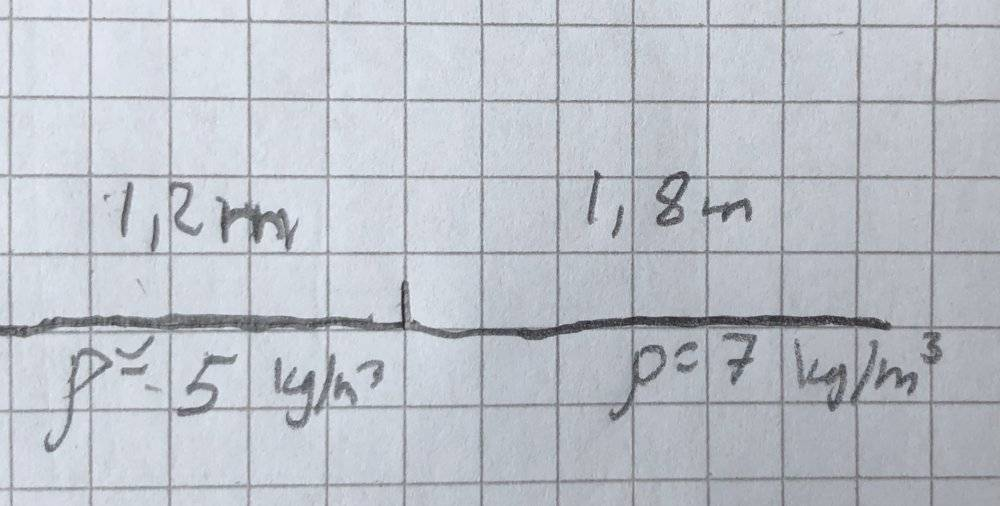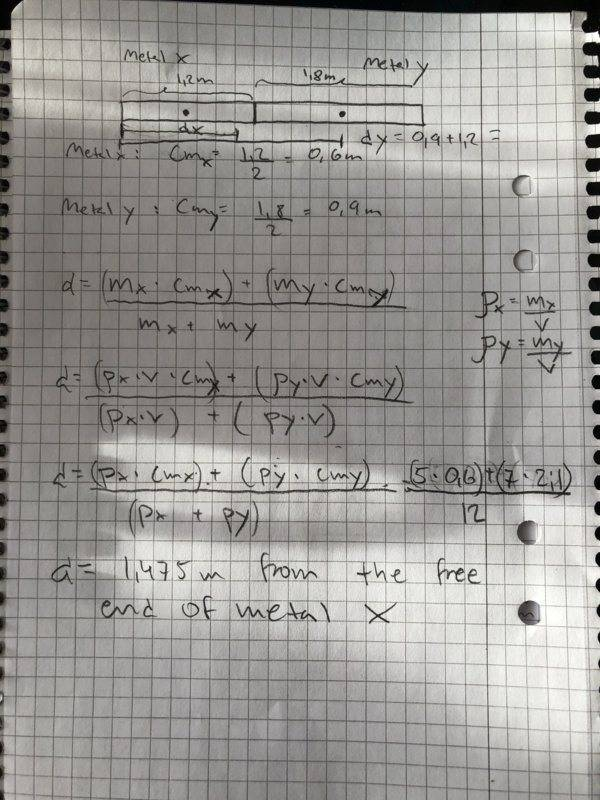# Calculate the center of mass of a non-uniform metal rod

VitaminK
Homework Statement:
A metal rod consists of two parts. one part is a 1.2m metal with the density of 5kg/m3. The other part is a 1,8m metal with the density of 7kg/m3. Calculate the center of mass for this rod.
Relevant Equations:
Density=m/vI know that if they had the same density they would have the center of mass at 1,5 m. But now that they don't the center of mass will be shifted towards the part of the rod with higher density. they will have their center of mass where they
have equal mass
p1*v=p2*v

now i don't know how to move forward

Gold Member
2022 Award
calculate the COM for each side then treat it as a 2-body system.

•FactChecker
VitaminK
Do you mean
1.2/2=0.6m
1.8/2= 0.9m
(0.6m+0.9m)

im not familiar with 2-body system (high school physics)

Gold Member
2022 Award
If you have a 100lb man standing at -10 on the x axix and a 200lb man standing at +10 on the x axis, where is the center of gravity of the two men considered as one system?

VitaminK
300lb/20 = 15 ?

Homework Helper
Gold Member
2022 Award
Do you mean
1.2/2=0.6m
1.8/2= 0.9m
(0.6m+0.9m)

im not familiar with 2-body system (high school physics)
Alternatively, try to find the point ##x## along the rod where there is equal mass on both sides.

PS Ignore this post!

Last edited:
VitaminK
Alternatively, try to find the point ##x## along the rod where there is equal mass on both sides.

you mean p1*v=p2*v --> 5*pi*r^2*l=7*pi*r^2*l now I am confused about the length l

Homework Helper
Gold Member
There are rod two parts, each with a uniform density, where the center of mass of each part is simple to determine. The original problem can be converted to a problem with the entire mass of the two parts located at their individual centers of mass. That problem is then simple to solve.

Homework Helper
Alternatively, try to find the point ##x## along the rod where there is equal mass on both sides.
That won't work. You are not after the point where the mass on both sides is the same. You need the leverage of the two masses to be the same.

Worded differently, you are not after the median [mass-weighted] position. You are after the mean [mass-weighted] position.

•PeroK
VitaminKIs this correct?

Homework Helper
Gold Member
2022 Award
you mean p1*v=p2*v --> 5*pi*r^2*l=7*pi*r^2*l now I am confused about the length l
Ignore what I said! I wasn't thinking.

Homework Helper
Gold Member
2022 Award
View attachment 260260
Is this correct?
That doesn't look right.

Can you break down your calculation so we can see where you've gone wrong?

Last edited:
•